We began our study of the shape of the universe by studying the shape of the Earth. Throughout these next few lessons it will sometimes be necessary to look to models or approximations to help us understand the characteristics of the universe we live in because many high school students have difficulty comprehending a space with more than three dimensions. We have been able to successfully measure the circumference and therefore the radius of the Earth. This demonstrates both how big, in comparison with our everyday lives and how small in comparison with the universe that the Earth really is. If we think more generally, the radius of the Earth also tells us something about its geometry. The Earth's radius is a length that best describes the rate of curvature of the surface of the Earth. For this reason, we can call it a radius of curvature. The radius is the important factor in the circumference, surface area and volume of the Earth. It is important to understand the role of the radius in an easily understandable situation since this concept will reappear when we study the curvature of the universe. What causes the spherical shape of the Earth? One of the most obvious causes affects our daily lives and holds us to the Earth's surface; it is called Gravity. Gravity can be thought of as a force which pulls every object that has mass towards all the other masses. For this reason, every part of the Earth pulls every other part of the Earth towards itself. In addition, the force of Gravity is inversely proportional to distance2, which means that the closer two masses are the harder they pull towards one another. Therefore if you add up all of these forces, which pull the parts of the Earth together, the net result is that all of the Earth's matter is pulled into a sphere. The shape of the Earth is not quite so simple, however. Other forces contribute to the shape of the Earth. Some obvious contributions to the imperfections of the Earth's spherical shape come from the plate tectonics of the Earth which form mountains. In comparison with the Earth's size, however, these "bumps" are truly insignificant. Less obvious is the bulge towards the Earth's Equator which is created by centrifugal force. As the Earth spins on its axis, the fastest moving parts, the parts at the equator, get pulled out away from the center of the Earth, making the slightly bulged or ellipsoidal shape. The two equations, which must balance against each other on every part of the Earth, are gravity which pulls inwards and centrifugal force which pulls outwards. Their equations are: Force of Gravity: F=(GM1m2)/RE2 Where G = a constant (6.67 x 10 -11m3/s/kg) m1, m2 are masses which attract each other RE is the Radius of the Earth And Centrifugal Force: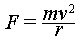Where m is the mass of the rotating body, V is the velocity the rotating part has R is the distance from the axis of rotation. How does this relate to the Universe? The shape of the observable universe, the part we can see, is a sphere. To understand why the observable universe is a sphere and exactly how big it is we need to go far back in the history of the universe. In the Expanding Universe section, we discovered that at some time in the distant past the universe was much hotter and denser than it is now. The evidence for this description of the early universe came from the supernova data, which showed that the farther away an object was from our point of observation, the faster it was travelling away from us. This meant that if all of the objects we could see were travelling away from us at some speed, then at some point in the past they must have been closer to us, resulting in a hotter and more dense universe. We used this evidence to approximate the age of the universe. Knowing that the slope of the line created in our Hubble diagram was in units of 1/time and calling it the Hubble Constant, we took the reciprocal of h0 to find the time that has elapsed since this hotter denser state. We decided that this represented the age of the Universe. We calculated this length of time to be approximately 15 billion years. This length of time is important because it regulates the size of the observable universe. Imagine the Earth at the center of a very large sphere. Imagine lots of stars on the outside surface of this sphere and nothing in between the stars and the Earth. We know from studying the speed of light that the distance to the surface of the sphere could be calculated from the speed of light and the length of time it took the light to reach Earth from the stars. In a similar manner, if we knew that certain light sources we could see were 15 billion light years away, then we know that we are seeing them as they were 15 billion years ago and that it took the light that long to travel to us. Because this could happen in any direction, we know that there is a sphere 15 billion light years away which is the farthest distance we can see. This is known as the observable universe. The only reason that this sphere is not any bigger is that the light has not had enough time since the beginning of the universe to reach the Earth. This sphere is, of course, expanding all of the time since with each second the universe gets one second older and light can travel one light second farther. In reality, we cannot always see right to the earliest parts of the universe. Lots of things get in the way and block our view of the universe, such as giant dust clouds and lots of other galaxies. The shape of the observable universe does not tell us the whole story, however. The universe as a whole could have many different shapes, each of which have different properties. One of the most important properties is called curvature. The Earth, which is a sphere-like shape, has positive curvature. There are also possibilities for the shape of the universe that have negative curvature. We will explore these shapes in the next activity, but first we must examine what might cause these different shapes. The Earth's shape was determined primarily by the force of gravity, so it is reasonable to think that gravity may be the cause of the shape of the universe. Scientists have discovered that gravity is also responsible for holding together the powerful explosions that heat the stars and for holding together the galaxies of stars and clusters of galaxies. For this reason, perhaps we should examine gravity as the primary force which shapes the universe. Since gravity is a force which is dependent both on mass and distance, we can easily study it by examining the density of the universe, which is a quantity with units of mass/distance3. Imagine there is a fixed amount of mass in the universe and a fixed volume. The density of the universe would therefore equal mass/volume. If gravity were the only force controlling this mass, then gravity would pull everything in this volume together and form a very dense ball of mass. So, why is the universe in which we live so spacious a place? There must be something which opposes this gravitational attraction. To discover what opposes gravity, we must again look to the expansion that we uncovered from the supernova lab. Whatever is causing this expansion must be opposing gravity. We can think of the balancing of these factors in this way. Imagine that you are standing on Earth and throwing a ball in the air. You give the ball some kinetic energy and as it rises in the air this energy is converted to gravitational potential energy. Eventually all of the kinetic energy is converted and the ball has a velocity of zero and then the potential energy begins to convert back into kinetic energy and the ball returns to Earth. You could imagine a situation where this is not the case, however. Imagine that you threw the ball so hard that the Earth's gravity could not pull the ball back towards itself and instead the ball kept going into space. This is the situation that could happen if you threw a ball at the Earth's escape velocity. You could also imagine a situation in the middle of these two extremes: you threw the ball just hard enough that it will not escape the Earth's gravity and yet it will not return to the Earth's surface. This situation is called orbit. When a shuttle orbits the Earth, it has just enough energy to escape falling back to Earth, but not enough to completely escape the Earth's gravitational pull and therefore it remains balanced in orbit. In the case of the universe, let's try to define the expansion and contraction factors that are opposing one another in terms of energy. First we have the kinetic energy from the expansion of the universe. If we assume that we are calculating the relationship of these energies in spherical shells then the kinetic energy from the expansion of one of these shells is: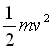where m is the mass of the shell and v is the velocity due to the expansion. The gravitational potential energy due to one of these shells is �Gmm/r, where G is the gravitational constant as before, m is the mass of the shell as above, r is the distance from the center of the shell to the surface of the shell, and m is the mass of all the stuff inside the shell. This mass can be calculated from the density (d) inside the shell times the volume inside the shell:. The total energy of the shell equals:If we substitute in v=Hr which we learned from the Hubble diagram in the Supernovae Lab Activity and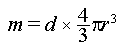from above this expression becomes: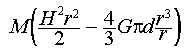Since the mass of this shell is constant and so is the energy we can divide out some of the constants and substitute k for (the total energy of the shell * (2/m)) to get the equation: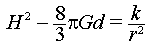This equation was discovered independently by Alexander Friedmann and Georges Lemaitre. What this equation tells us is exactly what we learned from the ball analogy: there is a balance between two types of energy and for differing values of d there will be very different outcomes. Remember the ball could fall back to Earth, fly far away from Earth or balance in between. For our equation of the universe, we will call the value of d which just balances the equation d(critical):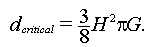In this case H equals the Hubble constant that we calculated using the Hubble Diagram. To date, scientists have tried many methods to calculate the actual value of the density of the universe without reaching a consensus. We are therefore unsure what will happen to the universe, but there are three possible scenarios: The density is more than d(critical): The universe will one day reverse direction and collapse back on itself, The density is exactly critical: The universe will just barely expand forever, The density is less than critical: The universe will continue to expand forever. We began writing these equations to see how gravity affects the shape of the universe. So what does this equation mean in terms of the shape of the universe? In order to understand this we must look to one of the most well known and respected scientists, Albert Einstein. Einstein reinterpreted the constant k from the above equation. We had said that k was a value for energy. Einstein instead interpreted it as a measure of the curvature of space. In the simplest of terms Einstein rewrote this equation as : "Matter tells space how to curve, and space tells matter how to move," (this description of Einstein's interpretation comes from John Wheeler's Gravitation). In other words, the curvature of space, like the curvature of the Earth is directly linked to the gravity which tells matter how to move. We can also reinterpret our three scenarios to describe the curvature of the universe, Density is more than critical: The universe will eventually re-collapse, k=+1 and the curvature is considered positive. This means it is like the surface of the Earth. Density is exactly critical: The Universe will just barely expand forever, k=0 and the curvature is considered flat. This means it is a plane just as in Euclidean geometry. Density is less than critical: The universe will expand forever, k=-1 and the curvature is considered negative. This means the surface is hyperbolic or saddle shaped. You can most easily think of a hyperbolic space in two dimensions as a rubber sheet which has two opposing corners pulled up and the other two corners pulled down, or the basic shape of a horse saddle. In the next activity we will examine more closely what it means for a surface to have negative or positive curvature; however, let's first consider the following example. Imagine two laser beams which are aligned completely parallel on a flat surface. If the beams continue forever without being interrupted, the beams will never intersect. This is one of the definitions students may learn in regular geometry class. Now if you put these beams on a spherical surface, like that of the Earth, and made the condition that the beams had to stay on the surface then the beams behave differently. On a spherical surface, beams (or straight lines) which start out parallel can intersect. Think of lines of longitude. They are parallel at the Equator and intersect at the poles. Now imagine a surface on which the parallel lines do not remain parallel and do not intersect but actually diverge from one another. One surface which has this property is called hyperbolic.Back | Next